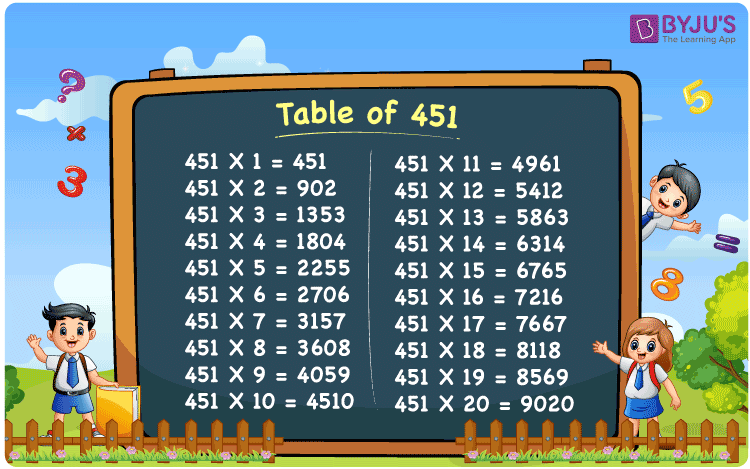Checkout JEE MAINS 2022 Question Paper Analysis : Checkout JEE MAINS 2022 Question Paper Analysis :

# Table of 451

The table of 451 is provided here, in different formats to help the students learn quickly. Pupils must memorise the table since it will aid in quick calculations. We have designed a table for 451 below, which will be useful in time-based examinations. For example, when 451 is multiplied by 3, the output is 451 x 3 = 451 + 451 + 451 = 1353. Similarly, we can get different values when 451 is multiplied by different integers.

## Table of 451 Chart## What is the 451 Times Table?

To obtain the 451 times table, we can simply add 451 to itself repeatedly in a sequence, as shown below.

 Using multiplication Using repeated addition 451 × 1 = 451 451 451 × 2 = 902 451 + 451 = 902 451 × 3 = 1353 451 + 451 + 451 = 1353 451 × 4 = 1804 451 + 451 + 451 + 451 = 1804 451 × 5 = 2255 451 + 451 + 451 + 451 + 451 = 2255 451 × 6 = 2706 451 + 451 + 451 + 451 + 451 + 451 = 2706 451 × 7 = 3157 451 + 451 + 451 + 451 + 451 + 451 + 451 = 3157 451 × 8 = 3608 451 + 451 + 451 + 451 + 451 + 451 + 451 + 451 = 3608 451 × 9 = 4059 451 + 451 + 451 + 451 + 451 + 451 + 451 + 451 + 451 = 4059 451 × 10 = 4510 451 + 451 + 451 + 451 + 451 + 451 + 451 + 451 + 451 + 451 = 4510

## Multiplication Table of 451

Find the multiplication table of 451 up to 20 results in the table given below.

 451 × 1 = 451 451 × 2 = 902 451 × 3 = 1353 451 × 4 = 1804 451 × 5 = 2255 451 × 6 = 2706 451 × 7 = 3157 451 × 8 = 3608 451 × 9 = 4059 451 × 10 = 4510 451 × 11 = 4961 451 × 12 = 5412 451 × 13 = 5863 451 × 14 = 6314 451 × 15 = 6765 451 × 16 = 7216 451 × 17 = 7667 451 × 18 = 8118 451 × 19 = 8569 451 × 20 = 9020

## Example on the Table of 451

Question:

John purchased a book at Rs. 451. Find the total cost if he bought twenty such books.

Solution:

The cost of 1 book = Rs. 451

Number of books bought by John = 20

Total cost = ?

Using the table of 451, 451 × 20 = 9020.

Therefore, the total cost of 20 books = Rs. 451 × 20 = Rs. 9020.

## Frequently Asked Questions on Table of 451

### What is the table of 451?

The table of 451 is also called the multiplication table of 451 or the 451 times table. Some of the results of the table of 451 are given below.
451 × 1 = 451
451 × 2 = 902
451 × 3 = 1353
451 × 4 = 1804
451 × 5 = 2255

### What is 451 times 6 plus 100?

451 times 6 plus 100 = 451 × 6 + 100 = 2706 + 100 = 2806

### 451 times of which number is equal to 6314?

451 times of fourteen, i.e. 451 times 14 = 451 × 14 = 6314.